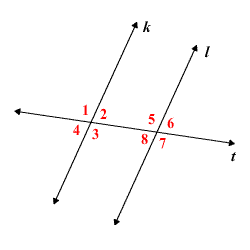# Alternate Interior Angles

The term alternate interior angles is often used when two lines are cut by a third line, a transversal .In the figure above, line $t$ is a transversal cutting lines $k$ and $l$ , and there are two pairs of alternate interior angles:

$\angle 2$ and $\angle 8$

$\angle 3$ and $\angle 5$

The Alternate Interior Angles Theorem states that if $k$ and $l$ are parallel , then the pairs of alternate interior angles are congruent . That is,

$\angle 2\cong \angle 8$ and $\angle 3\cong \angle 5$ .

The converse of this theorem is also true.